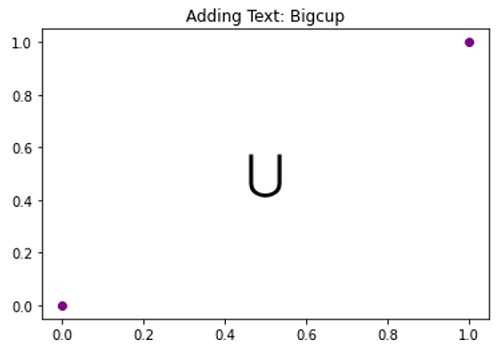# Bigcup Symbol in Python Plotting

Here, we are going to learn about the bigcup symbol in Python plotting and its implementation.
Submitted by Anuj Singh, on August 31, 2020

Bigcup is a different symbol used in set theory and python has provided us to use it while plotting. Following example shows its implementation.

```plt.text(0.45, 0.45, r'\$\bigcup\$')
```

Illustrations:## Python code for bigcup symbol in plotting

```import numpy as np
import matplotlib.pyplot as plt

# Bigcup
# In text
plt.figure()
plt.plot([0,1],[0,1], 'o', color=  'purple')
plt.text(0.45, 0.45, r'\$\bigcup\$', fontsize=25, )
plt.show()
```

Output:

```Output is as Figure
```

Languages: » C » C++ » C++ STL » Java » Data Structure » C#.Net » Android » Kotlin » SQL
Web Technologies: » PHP » Python » JavaScript » CSS » Ajax » Node.js » Web programming/HTML
Solved programs: » C » C++ » DS » Java » C#
Aptitude que. & ans.: » C » C++ » Java » DBMS
Interview que. & ans.: » C » Embedded C » Java » SEO » HR
CS Subjects: » CS Basics » O.S. » Networks » DBMS » Embedded Systems » Cloud Computing
» Machine learning » CS Organizations » Linux » DOS
More: » Articles » Puzzles » News/Updates